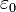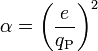## Tuesday, May 19, 2009

### Fine Structure Constant alpha = 1/137

From the Wiki entry on The Fine-Structure Constant:

As a dimensionless constant which does not seem to be directly related to any mathematical constant, the fine-structure constant has long fascinated physicists. Richard Feynman, one of the founders of quantum electrodynamics, referred to it in these terms:

“ It has been a mystery ever since it was discovered more than fifty years ago, and all good theoretical physicists put this number up on their wall and worry about it. Immediately you would like to know where this number for a coupling comes from: is it related to π or perhaps to the base of natural logarithms? Nobody knows. It's one of the greatest damn mysteries of physics: a magic number that comes to us with no understanding by man. You might say the 'hand of God' wrote that number, and 'we don't know how He pushed his pencil.' We know what kind of a dance to do experimentally to measure this number very accurately, but we don't know what kind of dance to do on the computer to make this number come out, without putting it in secretly! ”

—Richard P. Feynman (1985), QED: The Strange Theory of Light and Matter, Princeton University Press, p. 129, ISBN 0691083886

Three standard and equivalent definitions of α in terms of other fundamental physical constants are:where:is the elementary charge;is the reduced Planck constant;is the speed of light in vacuum;is the electric constant;is the magnetic constant or vacuum permeability, a defined conversion factor;is the constant of Coulomb's law.

The fine structure constant α has several physical interpretations.

In electrostatic cgs units, the unit of electric charge, the statcoulomb, is defined so that the permittivity factor, 4πε0, is 1 and dimensionless. The fine-structure constant then becomesPHYSICAL INTERPRETATIONS

* The square of the ratio of the elementary charge to the Planck charge* The ratio of two energies: (i) the energy needed to overcome the electrostatic repulsion between two electrons when the distance between them is reduced from infinity to some finite d, and (ii) the energy of a single photon of wavelength λ = 2πd (see Planck relation):* The ratio of the velocity of the electron in the Bohr model of the atom to the speed of light. Hence the square of α is the ratio between the electron rest mass (511 keV) and the Hartree energy (27.2 eV = 2 Ry).

* In quantum electrodynamics, α is the coupling constant determining the strength of the interaction between electrons and photons. The theory does not predict its value; therefore it must be determined experimentally. In fact, α is one of the ~20 empirical parameters in the Standard Model of particle physics, whose value is not determined within the Standard Model.

* In the electroweak theory unifying the weak interaction with electromagnetism, α is absorbed into two other coupling constants associated with the electroweak gauge fields. In this theory, the electromagnetic interaction is treated as a mixture of interactions associated with the electroweak fields. The strength of the electromagnetic interaction varies with the strength of the energy field.

When perturbation theory is applied to quantum electrodynamics, the resulting perturbative expansions for physical results are expressed as power series in α. Because α is clearly less than 1, higher powers of α are soon unimportant, making perturbation theory practical. In contrast, the large value of the corresponding factors in quantum chromodynamics makes calculations involving the strong force extremely difficult.

According to the theory of the renormalization group, the value of the fine structure constant (the strength of the electromagnetic interaction) grows logarithmically as the energy scale is increased. The observed value of α is associated with the energy scale of the electron mass; the electron is a lower bound for this energy scale because it (and the positron) is the lightest charged object whose quantum loops can contribute to the running. Therefore 1/137.036 is the value of the fine structure constant at zero energy. Moreover, as the energy scale increases, the strength of the electromagnetic interaction approaches that of the other two fundamental interactions, a fact important for grand unification theories. If quantum electrodynamics were an exact theory, the fine structure constant would actually diverge at an energy known as the Landau pole. This fact makes quantum electrodynamics inconsistent beyond the perturbative expansions.

The mathematician James Gilson has suggested  that the fine-structure constant has the value:29 and 137 being the 10th and 33rd prime numbers. The difference between the 2007 CODATA value for α and this theoretical value is about 3 x 10^-11, about 6 times the standard error for the measured value.## Friday, May 8, 2009

### 9. Octagonal Non-Space

It's funny where theory takes us. Based on the simplest assumptions combined with the avoidance of the human psychological drawback of the "double-blind" technique, theories take us where they will, sometimes into unexpected territory. If the conclusions based on the assumptions plus logic are proven incorrect, then the assumptions themselves are in doubt, and must be adjusted accordingly. This is where an Engineer has a slight advantage over a Mathematician or Physicist: We are used to iteration, to go either through, around, or over or under a wall rather than letting a wall stop us, whereas say a Physicist, such as De Broglie, is inclined to scrap promising ideas, such as his Pilot Wave Theory, if the results don't look promising.

I should mention before we go further that I do not believe in wielding Occam's Razor so much as wielding Occam's SWORD. A good sword too, quite sharp, and made of the finest of Arabian steel. However, the whole Occam thing is sorely misunderstood. Most interpret it to mean "the simplest solution is the best solution," but William of Occam never said that! What he did say in essence was "do not multiply a thing unnecessarily," meaning do not over-complicate a thing. That doesn't mean "simplest." It could mean the 2nd simplest solution, especially if the simplest solution is not backed up by experimental evidence.

What I presented in 7. The Tessellation Problem is one example. It would be nice if you could tessellate, or tile, three dimensions with tetrahedrons, but we cannot. The next simplest solution would be a combination of Tetrahedrons and Octagons.

(And yes, Cubes might seem to be simpler because they can tessellate space, but their structure is not nearly as simple as tetrahedrons. Also, I don't see cubes anywhere I look in the Universe, do you? My point being they are a way Euclid and Descartes have taught us to think mathematically, as x-,y-,and z- co-ordinates fit the way the human mind thinks best, but the Universe was here before we were.)

So what are these Octagons I propose? Are they "atoms of space" as the tetrahedrons are? Well, they could be, but then there would be two different "atom" shapes, which occurred to me to be unnecessarily complicated. Which means ...

Time to take out Occam's Sword! En gard !

Could these octagons be "non-space?" It would make more sense if they are. So what is "non-space?"

Before anyone submits the lame joke "non-sense," please hear me out.

I submit there are three kinds of space, the first of which we have never observed, and one I personally reject:

1) NEGATIVE SPACE - In such a space, if you took one step forward, you would end up one step back. This has never been observed outside of the U.S. Senate and the European Parliament, but those are macro-sized objects where quantum effects average out in the aggregate, so they don't count.

2) POSITIVE SPACE - This is the space we are all used to.

3) NON-SPACE - Alleged to exist within a wormhole, if you enter a non-space you appear instantaneously on the other side. It is as if space didn't exist! You are not traveling faster than the speed of light and thus breaking causality because the "space" you are going through doesn't exist! It's not even a "bubble" because "bubble" implies there is something inside.

In Pyron Theory the way such Octagonal non-spaces would work is that when a tetrahedral pyron faces an octahedral non-space non-pyron, the "pyronic energy" is instantly transferred from the pyramidal face of the sending tetrahedral pyron, "through" the non-space octagon, to the receiving triangular face opposite the sending one, instantaneously.

The mathematics continue to be developed and look promising when this approach is taken.